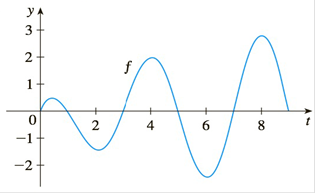Chapter 4.3, Problem 65E

Chapter
Section
Textbook Problem

# Let g ( x ) = ∫ 0 x f ( t )   d t where f is the function whosegraph is shown.(a) At what values of x do the local maximum and minimum values of g occur?(b) Where does g attain its absolute maximum value?(c) On what intervals is g concave downward?(d) Sketch the graph of g.To determine

a)

To find:

Values of x where local maximum and minimum occurs

Explanation

Concept:

i) If f changes from positive to negative at c, then f has a local maximum at c.

ii) If f changes from negative to positive at c, then f has a local minimum at c.

iii) If f is positive to the left and right of c or negative to the left and right of c, then f has no local maximum and minimum at c

Calculation:

By using the first fundamental theorem of calculus,

g(x)=f(x)

Therefore, the given graph is that of a derivative function

The local maxima and minima can be found where g(x) =f(x)=0, that is, where the graph meets the x axis

From the graph, the following conclusion can be made

On the interval (0, 1) graph is incre

To determine

b)

To find:

The absolute maximum value of g

To determine

c)

To find:

On what intervals is the curve concave downwards.

To determine

d)

To draw:

Graph of g

### Still sussing out bartleby?

Check out a sample textbook solution.

See a sample solution

#### The Solution to Your Study Problems

Bartleby provides explanations to thousands of textbook problems written by our experts, many with advanced degrees!

Get Started

#### Expand each expression in Exercises 122. (x+yxy)2

Finite Mathematics and Applied Calculus (MindTap Course List)

#### Find the mean for the following set of scores: 2, 7, 9, 4, 5, 3, 0, 6

Essentials of Statistics for The Behavioral Sciences (MindTap Course List)

#### The area of the shaded region is given by:

Study Guide for Stewart's Single Variable Calculus: Early Transcendentals, 8th